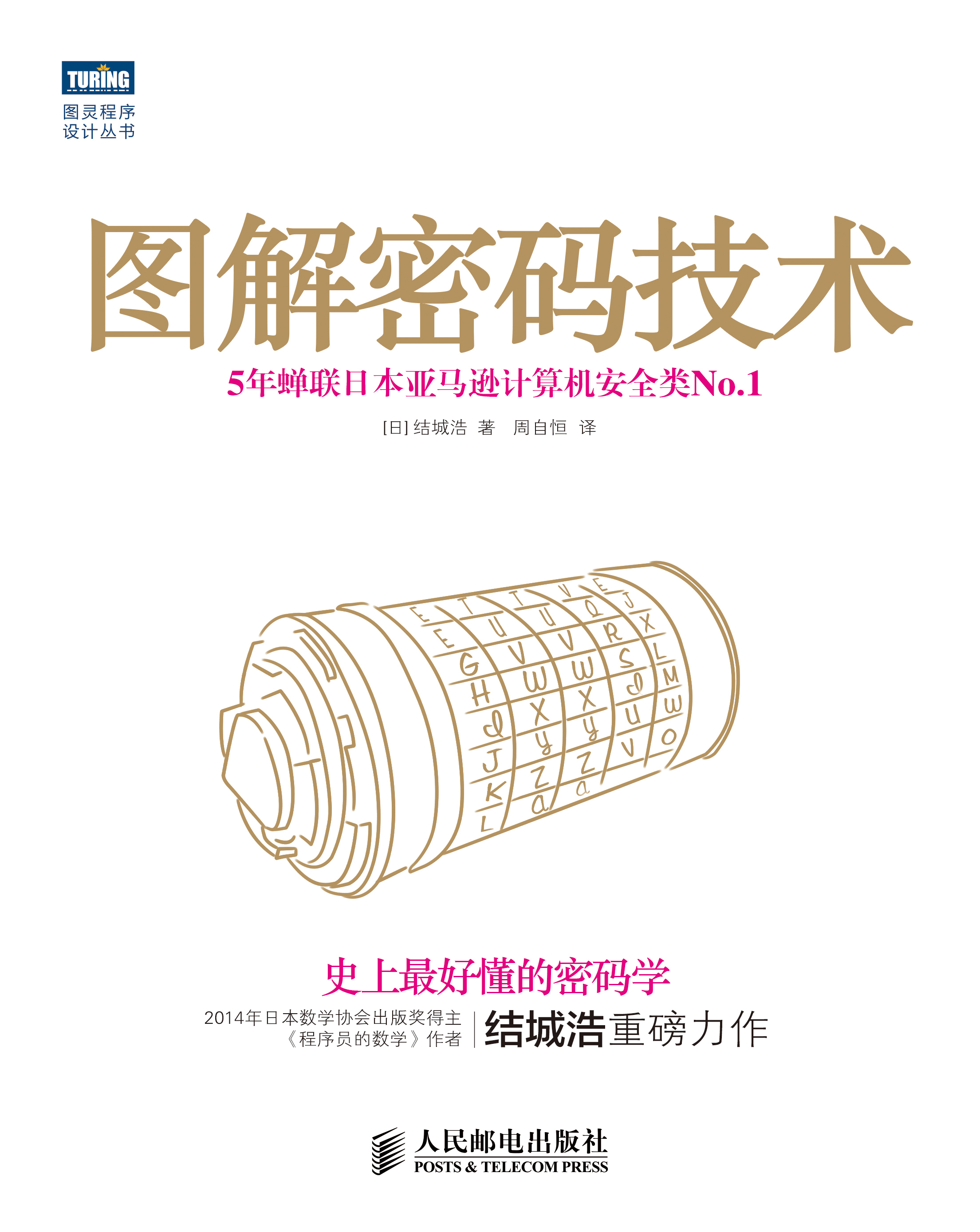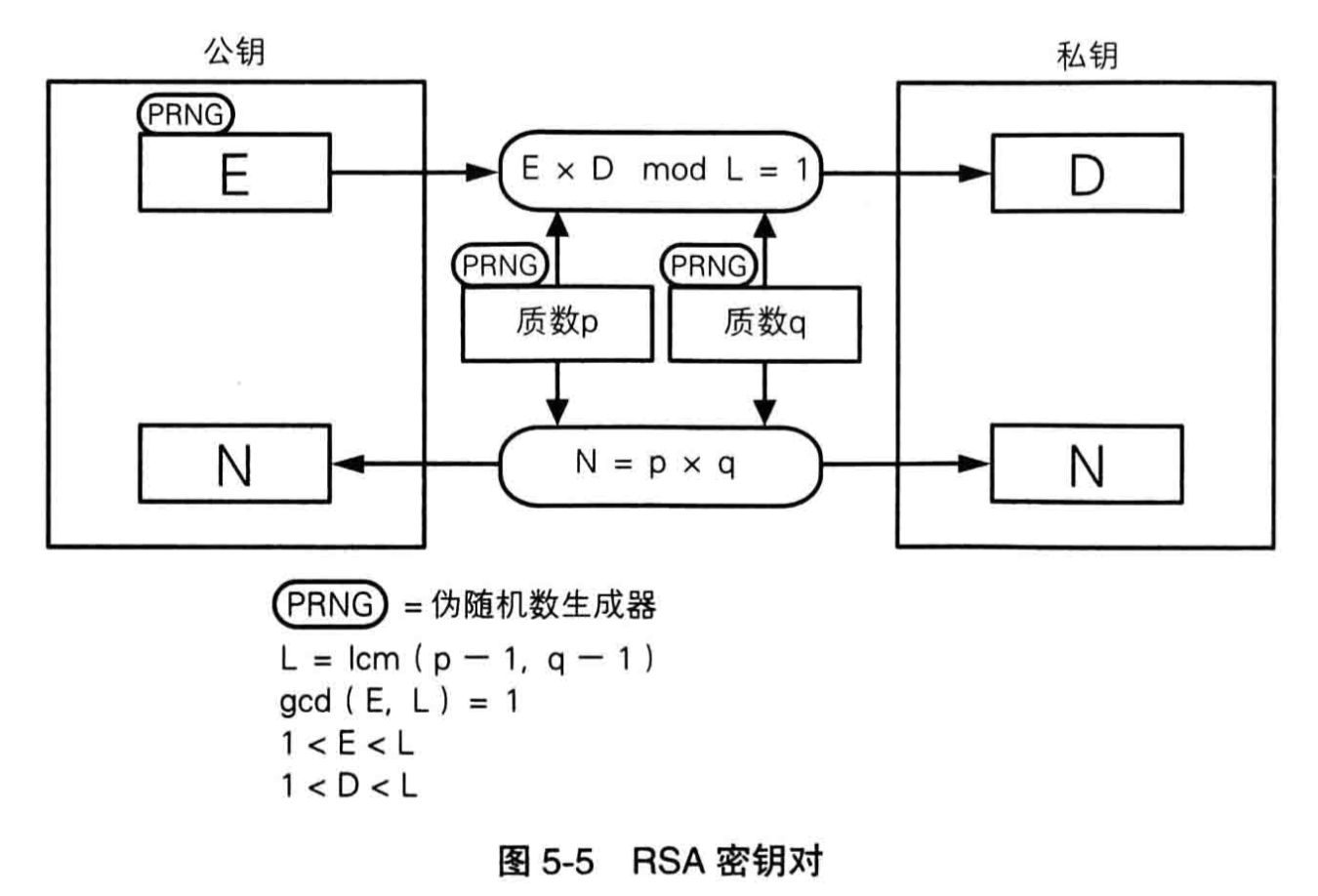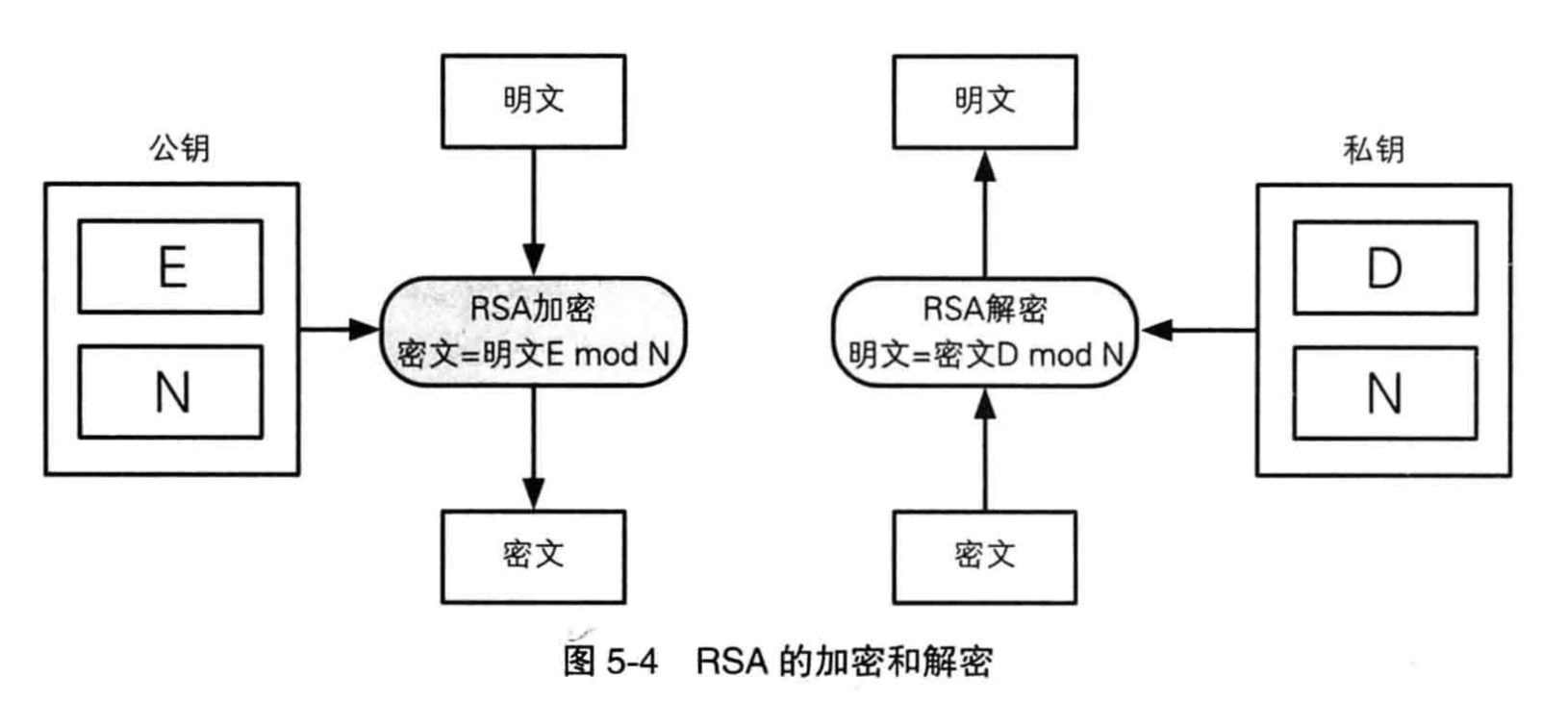# 手算RSA

RSA加密算法的地位无需赘述，其代码实现也多如牛毛，但徒手计算的能力仍然是有趣并有用的，这是内在修养。本文关注的是面向手工的计算方法，虽然拓展欧几里得算法不过三五行代码，但给你一支笔和一张纸，如何迅速地算出可靠结果呢？## 公钥(E,N)

p=17

q=19

E和L必须互质，且E<L。为了方便计算，就选最小的那个：E=5；于是公钥就选定了——(5,323)

## 私钥D

Ex+Ly=1；其中，x就是D，而y是与D相应的一个数，在RSA算法中并不关心。

 u' u x' x y' y q

### 填入初始状态

 u' u x' x y' y q 144 5 0 1 1 0

### 递推下一行

q=u'/u=144/5=28，余4。所以u'=u=5，而u=4。x = x' – xq = 0 – 1 * 28 = -28，y = y' – yq = 1 – 0 * 28 = 1。x' = x = 1, y' = y = 0。更新第二行：

 u' u x' x y' y q 144 5 0 1 1 0 28 5 4 1 -28 0 1

### 递推所有行

 u' u x' x y' y q 144 5 0 1 1 0 28 5 4 1 -28 0 1 1 4 1 -28 29 1 -1 4 1 0

5 * 29 + 144 * (-1) = 145 – 144 = 1 ，没有问题。### 评论 1

1. #1

表头写成L，E，D’ , D , Y’, Y ,C 更容易做考试题哈。

eric3年前 (2020-07-17)回复